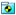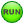# Exercise 1: Create and Use System Definitions

In this exercise, you will learn how to generate system definitions from the original system MDL file.

The exercise uses the following MDL statements:
• *DefineSystem()
• *System()
• *SetSystem()
• *Attachment()
You will complete these actions in this exercise:
• Modify the pendulum model from the tutorial MV-1060: Build a Pendulum Model Using MDL Statements to create a system definition called sys_pendu_mdl.
• Use this system definition to add another pendulum to the model and achieve the double pendulum model shown in Figure 1.
• Save the base model as doublependulum.mdl.
• Perform a dynamic simulation of the transient response and view the animation.

## Create a System Definition

In this step, you will create a system definition using the MDL model file from tutorial MV-1060: Build a Pendulum Model Using MDL Statements.

The structure of a system definition is similar to an MDL model file, so you can reuse the pendulum model file from the other tutorial to generate a more generalized system definition.
1. Copy the pendulum.mdl file, located in the mbd_modeling\mdl folder, to your <working directory>.
Below is a sample MDL file for the pendulum model in the previous tutorial:
//Pendulum Model
//05/31/XX
*BeginMDL(pendulum, "Pendulum Model")
//Topology information
//declaration of entities
//Points
*Point(p_pendu_pivot, "Pivot Point")
*Point( p_pendu_cm, "Pendulum CM")
//Bodies
//Graphics
*Graphic(gr_sphere, "pendulum sphere graphic", SPHERE, b_link,
p_pendu_cm, 1)
p_pendu_pivot, POINT, p_pendu_cm, 0.5, CAPBOTH)
//Revolute Joint
*RevJoint(j_joint, "New Joint", B_Ground, b_link, p_pendu_pivot,
VECTOR, V_Global_X)
//Output
*Output(o_pendu, "Disp Output", DISP, BODY, b_link)
//End Topology
// Property Information
*SetPoint(p_pendu_pivot, 0, 5, 5)
*SetPoint(p_pendu_cm, 0, 10, 10)
*SetBody( b_link, 1, 1000, 1000, 1000, 0, 0, 0)
*EndMDL()
You can convert the MDL file into a system definition by making small changes to the MDL file.
Important: This conversion is not applicable in all cases. You will need to take care of some conditions (which will be described later in the tutorial).
2. Replace the *BeginMDL() and *EndMDL() statements with the *DefineSystem() and *EndDefine() statements, respectively. Specify an appropriate variable name for the system definition.
3. Use the statements att_point and att_body to specify where the system connects (attachment point or pivot point) and to what body it connects (attachment body).
4. Use these variables in the *DefineSystem() statement.
*DefineSystem (sys_def_pendulum, att_point, att_body)
Note: As mentioned earlier, the attachment entity can be any MDL entity. Therefore you must specify the entity type that the variable represents (for example, att_point represents the POINT entity).
5. Use the *Attachment() statement to specify the entity type that each variable represents:
*Attachment (att_point, "Pivot Point", POINT, "Attachment point where
the pendulum definition gets attached")
*Attachment (att_body, "Attachment body" , BODY, " Any body to which the
pendulum definition gets attached")
Note: In the original model variable p_pendu_pivot represented the pivot point. While converting the pendulum model to pendulum system definition, this pivot point would be provided by the attachment point.
The point p_pendu_pivot is now passed as an attachment, therefore we do not need to define the pivot point.
6. Delete the statement *Point (p_pendu_pivot, "Pivot Point").
7. Retain the pendulum CM point, as well as the *Body() statement.
8. In the *RevJoint() statement, replace B_ground with att_body and replace p_pendu_pivot with att_point.
9. Retain the sphere *Graphic() statement.
10. In the cylinder *Graphic() statement, replace the variable p_pendu_pivot with att_point.
Note: Throughout the script, wherever applicable, the attachment variables should replace the original variables.
11. Retain the *Output() statement.
This allows you to obtain displacement outputs on each pendulum body in the model.
12. Remove *setpoint (p_pendu_pivot, 0, 5, 5).
13. In the *Setpoint() statement, parameterize the points in the system using the statement (att_point.y+5, att_point.z+5)
This will set the CM point 5 units away from the attachment point.
Note: Below is a sample system definition (system.mdl):
// system.mdl
// created on:
*DefineSystem(sys_def_pendulum, att_point, att_body)
//Topology Data
// Declaration of Entities
//Attachments
*Attachment (att_point, "Pivot Point", Point, "Attachment
point where the pendulum definition gets attached")
*Attachment (att_body, "Attachment body" , Body, " Any body
to which the pendulum definition gets attached")
//Points
*Point( p_pendu_cm, "Pendulum CM")
//Bodies
//Joints
*RevJoint(j_joint, "New Joint", att_body, b_link, att_point,
VECTOR, V_Global_X)
//Output
*Output(o_pendu, "Disp Output", DISP, BODY, b_link)
//Graphics
*Graphic(gr_sphere, "pendulum sphere graphic", SPHERE,
att_point, POINT, p_pendu_cm, 0.5, CAPBOTH )
// Property Data
*SetPoint(p_pendu_cm, 0, att_point.y+5, att_point.z+5)
*SetBody(b_link, 1, 1000, 1000, 1000, 0, 0, 0)
*EndDefine()

14. Save the file as sys_pendu.mdl.

In this step you will write an MDL file that includes the system definition and instantiate it multiple times.

1. In a text editor, create a new empty file.
2. Begin the model file with the *BeginMDL() statement.
3. From the sys_pendu.mdl file, copy the text from *DefineSystem() to *EndDefine() into the new file under the *BeginMDL() statement.
4. Use the *System() statement to instantiate the first pendulum system:
*System(system1, "First Pendulum System", sys_def_pendulum, P_Global_Origin, B_Ground)
Note: You can also refer to the MDL Language Reference online help for syntax.
Remember: When you instantiate the system, make sure to do the following:
• In the *System() statement, reference the system definition by specifying its variable name as the third argument. Use the same variable name for the system definition as you specified in the corresponding *DefineSystem() statement. In the above example, system1 uses the system definition sys_def_pendulum.
• If applicable, resolve any attachments in the system definition. For example, sys_def_pendulum has an attachment, att_body, to reference body_2 in the *RevJoint() statement. In system1, the pendulum body, b_link, should be connected to the ground body, B_Ground. Therefore, B_Ground is specified as the attachment body in the *System() statement.
• It is recommended that you add the *System() statement before *DefineSystem(), although it is not mandatory.
5. Repeat step 4 (with appropriate modifications) to create the second pendulum system.
1. Provide a different variable name, system2, for the system instance.
2. Use Pendulum CM (p_pendu_cm) and the Pendulum Body (b_link) from the first system as the attachment.
The exact statement you should use is shown below:
*System(system2, "Second Pendulum System", sys_def_pendulum,
system1.p_pendu_cm, system1.b_link )
6. Use the *EndMDL() statement to close the file.
An example of the model file is shown below:
*BeginMDL(model, "MODEL")
*System(system1, "First Pendulum System", sys_def_pendulum,
P_Global_Origin, B_Ground)
*System(system2, "Second Pendulum System", sys_def_pendulum,
*DefineSystem(sys_def_pendulum, att_point, att_body)
//Topology Data
// Declaration of Entities
//Attachments
*Attachment (att_point, "Pivot Point", Point, "Attachment point
where the pendulum definition gets attached")
*Attachment (att_body, "Attachment body" , Body, " Any body to which
the pendulum definition gets attached")
//Points
*Point( p_pendu_cm, "Pendulum CM")
//Bodies
//Joints
*RevJoint(j_pivot, " Revolute Joint at Pivot Point ", b_link,
att_body, att_point, VECTOR, V_Global_X)
//Output
*Output(o_pendu, "Disp Output", DISP, BODY, b_link)
//Graphics
*Graphic(gr_sphere, "pendulum sphere graphic", SPHERE, b_link,
p_pendu_cm, 1 )
att_point, POINT, p_pendu_cm, 0.5, CAPBOTH )
// Property Data
*SetPoint(p_pendu_cm, 0, att_point.y+5, att_point.z+5)
*SetBody(b_link, 1, 1000, 1000, 1000, 0, 0, 0)
*EndDefine()
*EndMDL()
7. Save the model as doublependulum.mdl.
8. Open the MDL file in MotionView. Review the model, focusing particularly on the First Pendulum System and Second Pendulum System files listed in the Project Browser.
Under the(System) icon, you will notice a small 'hand' symbol. This indicates a feature known as Shared Definition, where both systems have a single definition. When a system definition is shared, any modification to one instance can be made to reflect all instances.
9. Make modifications to all instances.
1. In the menu bar, click Tools > Options.
2. In the Options dialog, click Build Model (located near the bottom of the tree).
3. Under Legacy Support, uncheck the Create separate definitions when modifying a shared instance option.
Now when you make modifications to an instance, the change will be applied across all instances without creating a separate definition.
10. Click OK.
11. Run the MotionSolve simulation and post-process the results.
1. Click the(Run) panel button.
2. In the panel, specify an End time of 1.0 and a Print interval of 0.01.
3. Click the Run button.